• Call Now

1800-102-2727•

# <!--td {border: 1px solid #ccc;}br {mso-data-placement:same-cell;}--> Definition of Heat Capacity and Specific Heat Capacity: Heat Capacity at Constant Volume (CV) & Constant Pressure (Cp) Relation

Beaches are such a beauty of nature, aren’t they? This is really what we need when we are in the mood to relax. We love watching water meeting the sand and the sunset at the horizon.The sand on the beach is hot when the sun is beating down, but what about the water in the sea? Well! It's much cooler! Have you ever wondered why the water is cooler than the sand even though they are heated by the same source up there? Let’s look at what is happening here.

e and a wooden spoon in the second. The metal spoon gets heated quite quickly in terms of temperature rie. For the same amount of heat, the temperature of the metal spoon increases a lot in comparison with the wooden spoon. If we were to observe a one-degree temperature increase in the

## Introduction to Heat Capacity

Let’s assume that both the land surface near to the sea and water are at the same temperature, say 200C Both got exposed to the sun till noon, due to this the temperature of the land surface has been raised to 40oC but the temperature of the water is still pleasant, 25oC. We can see that despite being exposed to the same amount of heat, the rise in temperature of land and water is not the same.

Clearly, both have shown different sensitivities to heat in regards to temperature, isn’t it?

Let’s substantiate the above claim with some facts. It takes about 78,000 joules of heat to increase the temperature of a kilogram of seawater from 20oC to  40oC. Whereas it takes only about 15,000 joules for the same mass of sand to increase its temperature from 20oC to  40oC.

So we can clearly see that different substances require different amounts of heat to raise the temperature by the same amount. Alternatively, it can be said that some substances show a higher temperature rise than others for the same amount of heat they absorb. By knowing how much heat a given substance needs to raise its temperature by a particular amount is something of relevance to us in thermodynamics. The property that specifically tells us about this is called heat capacity.## Definition of Heat Capacity

The heat capacity of a substance can be defined as the amount of heat required to raise the temperature of a given amount of substance by one-degree celsius.

Mathematically, heat capacity can be expressed as: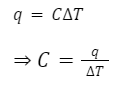Where,q is the heat energy required to bring about a temperature change of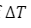and C is the heat capacity of the substance.

Similarly, it can be written as:

dq = cdT (for a very small change in the temperature)

Integrating both the sides,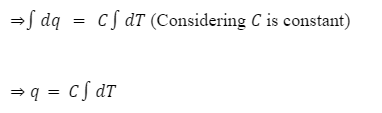Heat capacity for a given matter depends on its size or quantity and hence it is an extensive property. The unit of heat capacity isJ K-1 or J0C-1.

## Specific Heat Capacity (Cs)

Scientists defined specific heat capacity as a quantity that was independent of the quantity or size of the material being studied for thermodynamic investigations. Since it is unaffected by the size or quantity of the matter, it is an intensive quality.

Specific heat capacity of a substance can be defined as the amount of heat energy required to raise the temperature of one kilogram of a substance by one-degree celsius.

Mathematically, specific heat capacity can be expressed as: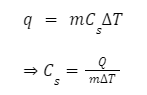Where Q is the amount of heat transfer,is the change in the temperature, m is the mass of the substance and Cs is the specific heat capacity.

The unit of specific heat capacity (CS) is K Kg-1K-1

Similarly it can be written as:

dq = mCsdT (for a very small change in the temperature)

Integrating both the sides,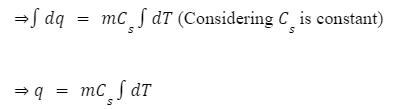## Molar Heat Capacity ((CM))

Molar heat capacity of a substance can be defined as the amount of heat energy required to raise the temperature of one mole of a substance by one-degree celsius.

Mathematically, molar heat capacity can be expressed as: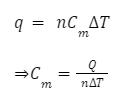Where Q is the amount of heat transfer,is the change in the temperature, n is the no. of moles of the substance and Cis the specific heat capacity.

Similarly it can be written as:

dq = nCmdT (for a very small change in the temperature)

Integrating both the sides,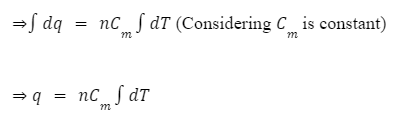The unit of Cm is J mol-1K-1

The molar heat capacity is an intensive property of a substance, that does not depend on the amount and size of the substance.

## Relation Between Specific Heat Capacity and Molar Heat Capacity

Molar heat capacity (Cm) is the product of the specific heat capacity (Cs) and the molar mass of the substance (M).

Mathematically,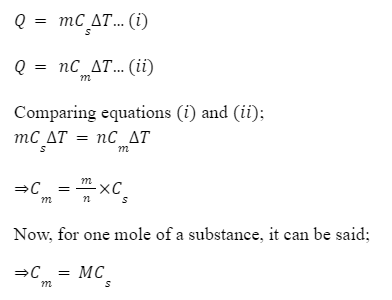Where, M is the molar mass of the substance

## Heat Capacity at Constant Volume (Cv)

The heat required to raise the temperature of one mole of gas by 1 °C (or 1 K) at constant

volume is called heat capacity at constant volume (Cv).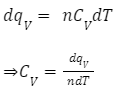Where, n is no. of moles of the gas, dqv is heat transfer at constant volume, dT is a small change in the temperature.

## Heat Capacity at Constant Pressure (Cp)

The heat required to raise the temperature of one mole of gas by 1 °C (or 1 K) at constant

pressure is called heat capacity at constant pressure (Cp).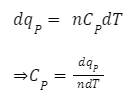Where, n is no. of moles of the gas, dpq is heat transfer at constant pressure, dT is a small change in the temperature.

## Cp-CvRelation

Consider an ideal gas. Let dq be the amount of heat given to the system to raise the temperature of an ideal gas by dT, and change in internal energy be du. Then, According to the first law of thermodynamics;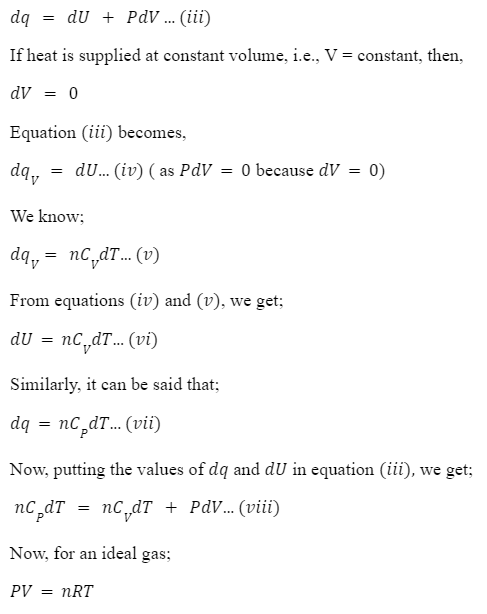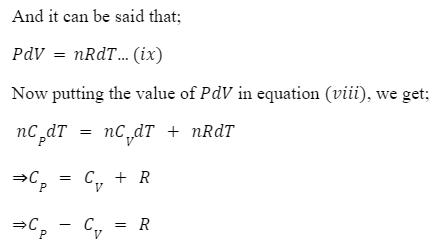Note: The above relation between Cp&Cv is true only for an ideal gas.

## Practice Problems on Heat Capacity

Q 1. (Cp - Cv) = R  is for n moles of a gas. If we consider 1 mol of the gas. The correct formula among the following is:

##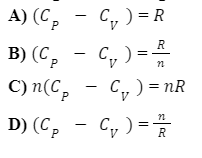We know;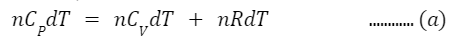Equation (a) is derived from the first law of thermodynamicsfor n moles of a gas.

If we consider  n = 1 mol putting the value of n in equation (a), we get;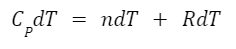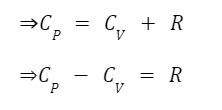Q 2.  The amount of heat required to raise the temperature of the unit mass of a gas by one-degree Celcius at constant pressure is:

A) Cv

B) Cp

C) Cs

D) None of the above

The heat required to raise the temperature of one mole of gas by 1 °C (or 1 K) at constant

pressure is called heat capacity at constant pressure (Cp).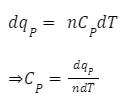Q 3.  10 g piece of a metal absorbs 300 J of energy in the form of heat. The temperature of the metal got raised from 270C to 470C.  The specific heat of the metal would be: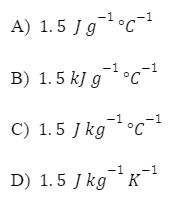Given,

q = 300 j

m = 10 g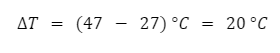We know that,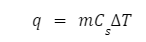Where,

q = heat energy,

Cs = specific heat capacity

m = ass of a substance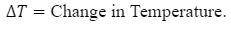Then, specific heat capacity,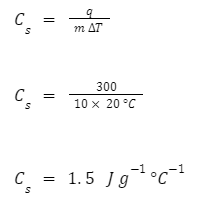Q 4.  550 j of heat was absorbed by a sample of CaCO3 when its temperature was increased by 5K . If the molar heat capacity of CaCO3 is 82 J mol-1K-1, then the number of moles of CaCO3 will be:

a. 2.50 mol

b. 3.45 mol

c.  1.34 mol

d.  1.70 mol

Given,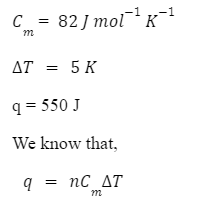Where,

q =  heat energy

Cm =  molar heat capacity

n = number of molesOn rearranging the equation, we get,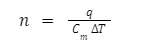Substituting the values in the equation, we get,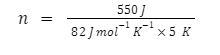n = 1.34 mol

## FAQ's on Heat Capacity

Q 1. Can the heat capacity of any substance be zero?

Answer: Zero heat capacity of any substance means an infinitesimally small amount of heat will increase the temperature by an infinitely large amount because it has very less internal degrees of freedom into which it can channel the absorbed energy or heat. The smaller the heat capacity, the lesser the number of internal degrees of freedom and vice versa.

Q 2. What is the importance of heat capacity?

Answer: Larger the heat capacity, the more the heat a substance can store, even with a slight increment in the temperature. For example; water has high heat capacity, so even to increase the slightest of temperature it would need a large amount of heat energy.

That’s why water's high heat capacity makes it ideal for central heating systems since it can transfer a lot of energy even with a slight change in temperature.

Q 3. Is specific heat capacity an intensive or extensive property?

Answer: Specific heat is an intensive property. Its magnitude does not depend upon the amount of matter present in the system.

Q 4. What factors affect heat capacity?

Answer: Experiments show that the transferred heat depends on the following factors:

• Change in temperature
• Amount of the substance
• Nature of the substance
• Phase of the substance

Q 5. How do we measure heat capacity?

Answer: Start with the object at a known uniform temperature, add a known amount of heat energy, wait for the temperature to become uniform, and measure the change in temperature and then by using the formula of heat transfer;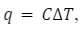, calculate the heat capacity of a substance (C).

## Related Topics:Talk to our expert
Resend OTP Timer =
By submitting up, I agree to receive all the Whatsapp communication on my registered number and Aakash terms and conditions and privacy policy